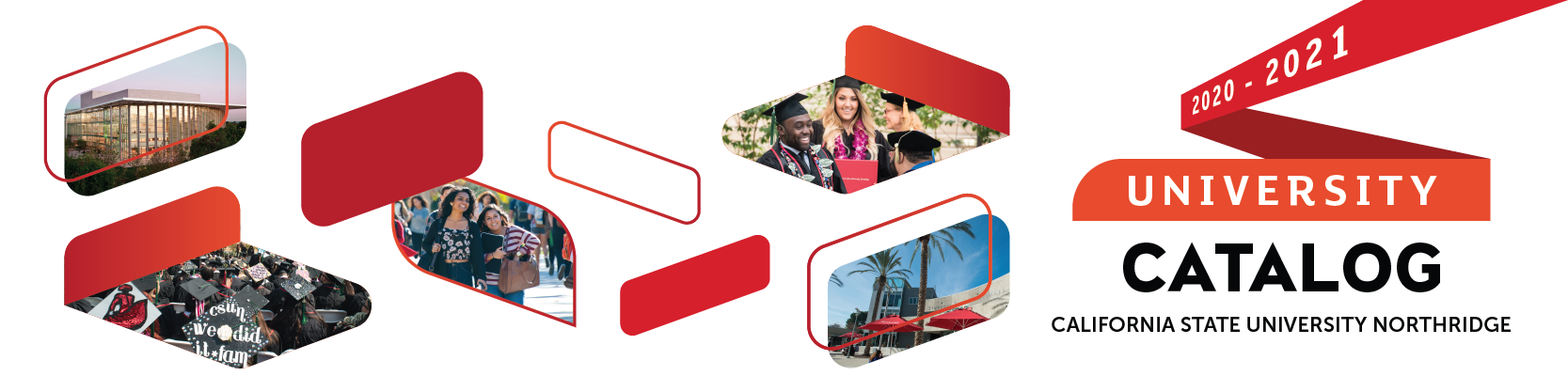## Course: MATH 462. Advanced Linear Algebra (3)

Prerequisites: MATH 262, MATH 320. Recommended Corequisite or Preparatory MATH 360. This course covers vector spaces and linear transformations from a more theoretical perspective than that covered in MATH 262. The course begins with a review of abstract vector spaces, including the invariance of dimension of a finite dimensional vector space, the correspondence between linear transformations and matrices, similarity, as well as the definition and basic properties of determinants. The concepts of eigenvalues, eigenvectors, and diagonalization will be reviewed. The course will also cover real and complex inner product spaces, Hermitian operators, the spectral theorem, minimal and characteristic polynomials, the Cayley Hamilton theorem, and the Jordan canonical form.

### MATH 462

Class NumberLocationDayTime